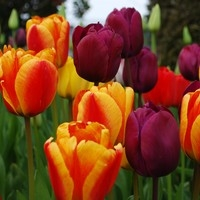# JB0O0MFavorite genre

Hiphop, R&B, Dance/Disco, Electronic/Techno, Pop, Ballad, Jazz, Latin, Classical/New Age, Soul/Funk/Groove

Favorite artists

v^v^v^v^v^vI don't have any yetv^v^v^v^v^v

^v^v^v^v^v^v^v^v^v^v^v^v^v^v^v^v^v^v^v^v^v^v^v^v^v^v^v^v^v^v^v^v^v^v^v^v
I don't have much to say but that is okay I guess XD
^v^v^v^v^v^v^v^v^v^v^v^v^v^v^v^v^v^v^v^v^v^v^v^v^v^v^v^v^v^v^v^v^v^v^v^v
Hello loved ones!~ JB0O0M here! My name is La'Jasha, I like to go by Jasha :3. I am 14 and African American born in East Texas. I been in the US for my whole life. I plan to travel when I am older, have time and money to see the world. SO let's start of with me bio. I like anime, drawing, dancing, gaming, sewing, funny stuff, etc.
^v^v^v^v^v^v^v^v^v^v^v^v^v^v^v^v^v^v^v^v^v^v^v^v^v^v^v^v^v^v^v^v^v^v^v^v
Music soothes, excites, and just gives me that funny bubbly feeling on the inside, sometimes it makes me wanna dance too. That is what I like about music. You can express yourself in music in many ways which makes the purpose of it so legit. I've joined MusicShake to share my creations and let the world around me listen. To let them know that I enjoy it and maybe they can too. The same thing goes for other music creators, I would love to hear what you make too. I mostly play video games and draw digital art (alot of anime XD) and stuff. What I eventually want to do for my career is become a video game designer. I find that the music gives the game a mood setting so that you can know it fully. Who knows, I may want to become a music producer too. Jpop, Kpop, Dub-step, little bit of R&B and remixes are my favorites.
^v^v^v^v^v^v^v^v^v^v^v^v^v^v^v^v^v^v^v^v^v^v^v^v^v^v^v^v^v^v^v^v^v^v^v^v
I'll be happy listening. Toodles~
^v^v^v^v^v^v^v^v^v^v^v^v^v^v^v^v^v^v^v^v^v^v^v^v^v^v^v^v^v^v^v^v^v^v^v^v
(I like bells ;3)
Keeping time, time, time,
In a sort of Runic rhyme,
To the tintinnabulation that so musically wells
From the bells, bells, bells, bells,
Bells, bells, bells -
From the jingling and the tinkling of the bells.